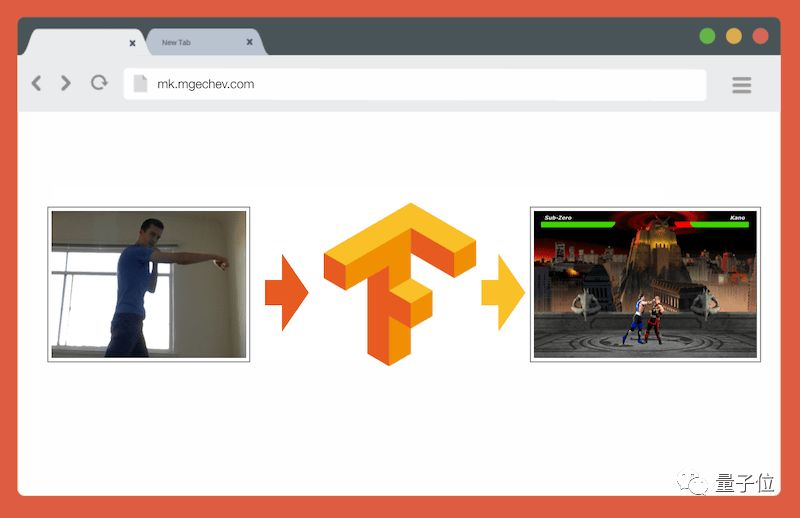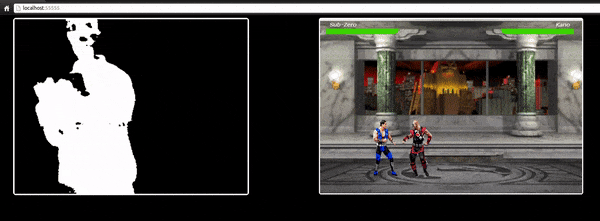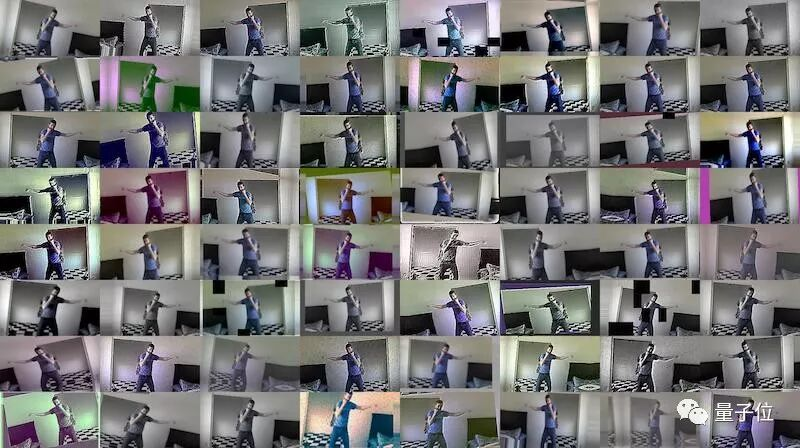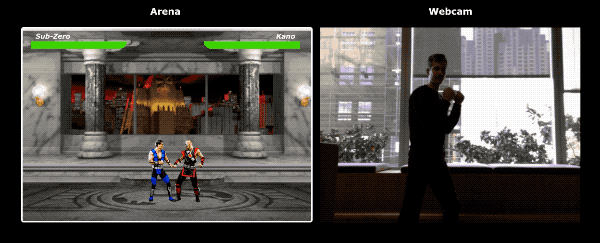# 笔记本玩体感游戏5年间，无论是网络浏览器的API，还是WebGL都有了长足的发展。于是这名工程师决定用TensorFlow.js来改进他的游戏程序，并在他个人Blog上放出了完整教程。

# 简介

• 为图片分类收集数据
• 使用imgaug进行数据增强
• 使用MobileNet迁移学习
• 二元分类和N元分类
• 在浏览器中使用TensorFlow.js模型训练图片分类# 收集数据

• 拳击
• 踢腿
• 其他

ffmpeg -i video.mov \$filename%03d.jpg# 数据增强

``````np.random.seed(44)
ia.seed(44)

def main():
for i in range(1, 191):
draw_single_sequential_images(str(i), "others", "others-aug")
for i in range(1, 191):
draw_single_sequential_images(str(i), "hits", "hits-aug")
for i in range(1, 191):
draw_single_sequential_images(str(i), "kicks", "kicks-aug")

def draw_single_sequential_images(filename, path, aug_path):
image = misc.imresize(ndimage.imread(path + "/" + filename + ".jpg"), (56, 100))
sometimes = lambda aug: iaa.Sometimes(0.5, aug)
seq = iaa.Sequential(
[
iaa.Fliplr(0.5), # horizontally flip 50% of all images
# crop images by -5% to 10% of their height/width
sometimes(iaa.CropAndPad(
percent=(-0.05, 0.1),
pad_mode=ia.ALL,
pad_cval=(0, 255)
)),
sometimes(iaa.Affine(
scale={"x": (0.8, 1.2), "y": (0.8, 1.2)}, # scale images to 80-120% of their size, individually per axis
translate_percent={"x": (-0.1, 0.1), "y": (-0.1, 0.1)}, # translate by -10 to +10 percent (per axis)
rotate=(-5, 5),
shear=(-5, 5), # shear by -5 to +5 degrees
order=[0, 1], # use nearest neighbour or bilinear interpolation (fast)
cval=(0, 255), # if mode is constant, use a cval between 0 and 255
mode=ia.ALL # use any of scikit-image's warping modes (see 2nd image from the top for examples)
)),
iaa.Grayscale(alpha=(0.0, 1.0)),
iaa.Invert(0.05, per_channel=False), # invert color channels
# execute 0 to 5 of the following (less important) augmenters per image
# don't execute all of them, as that would often be way too strong
iaa.SomeOf((0, 5),
[
iaa.OneOf([
iaa.GaussianBlur((0, 2.0)), # blur images with a sigma between 0 and 2.0
iaa.AverageBlur(k=(2, 5)), # blur image using local means with kernel sizes between 2 and 5
iaa.MedianBlur(k=(3, 5)), # blur image using local medians with kernel sizes between 3 and 5
]),
iaa.Sharpen(alpha=(0, 1.0), lightness=(0.75, 1.5)), # sharpen images
iaa.Emboss(alpha=(0, 1.0), strength=(0, 2.0)), # emboss images
iaa.AdditiveGaussianNoise(loc=0, scale=(0.0, 0.01*255), per_channel=0.5), # add gaussian noise to images
iaa.Add((-10, 10), per_channel=0.5), # change brightness of images (by -10 to 10 of original value)
iaa.AddToHueAndSaturation((-20, 20)), # change hue and saturation
# either change the brightness of the whole image (sometimes
# per channel) or change the brightness of subareas
iaa.OneOf([
iaa.Multiply((0.9, 1.1), per_channel=0.5),
iaa.FrequencyNoiseAlpha(
exponent=(-2, 0),
first=iaa.Multiply((0.9, 1.1), per_channel=True),
second=iaa.ContrastNormalization((0.9, 1.1))
)
]),
iaa.ContrastNormalization((0.5, 2.0), per_channel=0.5), # improve or worsen the contrast
],
random_order=True
)
],
random_order=True
)

im = np.zeros((16, 56, 100, 3), dtype=np.uint8)
for c in range(0, 16):
im[c] = image

for im in range(len(grid)):
misc.imsave(aug_path + "/" + filename + "_" + str(im) + ".jpg", grid[im])``````# 在浏览器中运行模型

``````const video = document.getElementById('cam');
const Layer = 'global_average_pooling2d_1';
const mobilenetInfer = m => (p): tf.Tensor<tf.Rank> => m.infer(p, Layer);
const canvas = document.getElementById('canvas');
const scale = document.getElementById('crop');

const ImageSize = {
Width: 100,
Height: 56
};

navigator.mediaDevices
.getUserMedia({
video: true,
audio: false
})
.then(stream => {
video.srcObject = stream;
});``````

• video：页面中的HTML5视频元素
• Layer：MobileNet层的名称，我们从中获得输出并把它作为我们模型的输入
• mobilenetInfer：从MobileNet接受例子，并返回另一个函数。返回的函数接受输入，并从MobileNet特定层返回相关的输出
• canvas：将取出的帧指向HTML5的画布
• scale：压缩帧的画布

``````const grayscale = (canvas: HTMLCanvasElement) => {
const imageData = canvas.getContext('2d').getImageData(0, 0, canvas.width, canvas.height);
const data = imageData.data;
for (let i = 0; i < data.length; i += 4) {
const avg = (data[i] + data[i + 1] + data[i + 2]) / 3;
data[i] = avg;
data[i + 1] = avg;
data[i + 2] = avg;
}
canvas.getContext('2d').putImageData(imageData, 0, 0);
};``````

``````let mobilenet: (p: any) => tf.Tensor<tf.Rank>;
tf.loadModel('http://localhost:5000/model.json').then(model => {
mobileNet
.load()
.then((mn: any) => mobilenet = mobilenetInfer(mn))
.then(startInterval(mobilenet, model));
});``````

``````const startInterval = (mobilenet, model) => () => {
setInterval(() => {
canvas.getContext('2d').drawImage(video, 0, 0);

grayscale(scale
.getContext('2d')
.drawImage(
canvas, 0, 0, canvas.width,
canvas.width / (ImageSize.Width / ImageSize.Height),
0, 0, ImageSize.Width, ImageSize.Height
));

const [punching] = Array.from((
model.predict(mobilenet(tf.fromPixels(scale))) as tf.Tensor1D)
.dataSync() as Float32Array);

const detect = (window as any).Detect;
if (punching >= 0.4) detect && detect.onPunch();

}, 100);
};``````

startInterval正是关键所在，它每间隔100ms引用一个匿名函数。在这个匿名函数中，我们把视频当前帧放入画布中，然后压缩成100*56的图片后，再用于灰阶滤波器。# 用N元分类识别拳击和踢腿

``````const punches = require('fs')
.readdirSync(Punches)
.filter(f => f.endsWith('.jpg'))
.map(f => `\${Punches}/\${f}`);

const kicks = require('fs')
.readdirSync(Kicks)
.filter(f => f.endsWith('.jpg'))
.map(f => `\${Kicks}/\${f}`);

const others = require('fs')
.readdirSync(Others)
.filter(f => f.endsWith('.jpg'))
.map(f => `\${Others}/\${f}`);

const ys = tf.tensor2d(
new Array(punches.length)
.fill([1, 0, 0])
.concat(new Array(kicks.length).fill([0, 1, 0]))
.concat(new Array(others.length).fill([0, 0, 1])),
[punches.length + kicks.length + others.length, 3]
);

const xs: tf.Tensor2D = tf.stack(
punches
.map((path: string) => mobileNet(readInput(path)))
.concat(kicks.map((path: string) => mobileNet(readInput(path))))
.concat(others.map((path: string) => mobileNet(readInput(path))))
) as tf.Tensor2D;``````# 动作识别• 自然语言处理，词语的意思需要联系上下文
• 根据历史记录，预测用户将要访问的页面
• 识别一系列帧中的动作

# 总结

https://github.com/mgechev/movement.js

JS版《真人快打》项目地址：
https://github.com/mgechev/mk.js

imgaug：
https://github.com/aleju/imgaug

MobileNet神经网络：
https://www.npmjs.com/package/@tensorflow-models/mobilenet